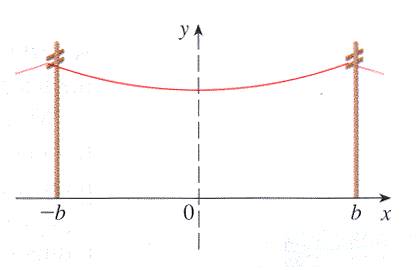The figure below shows a telephone wire hanging between two poles at $x=-b$ and $x=b.$ It takes the shape of a catenary with equation $y=c+a\cosh(x/a).$(a) Find the length of the wire.

Length of wire =

(b) Suppose two telephone poles are 50 ft apart and the length of the wire between the poles is 51 ft. If the lowest point of the wire must be 20 ft above the ground, how high up (in ft) on each pole should the wire be attached? Give your answer correct to two decimal places.

feet

You can earn partial credit on this problem.